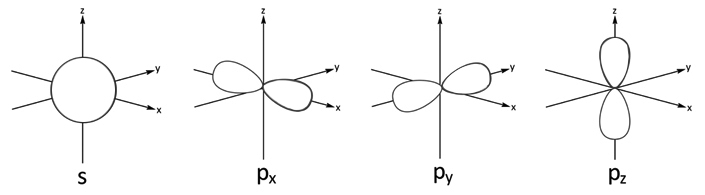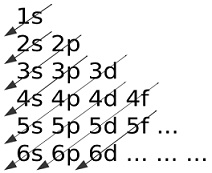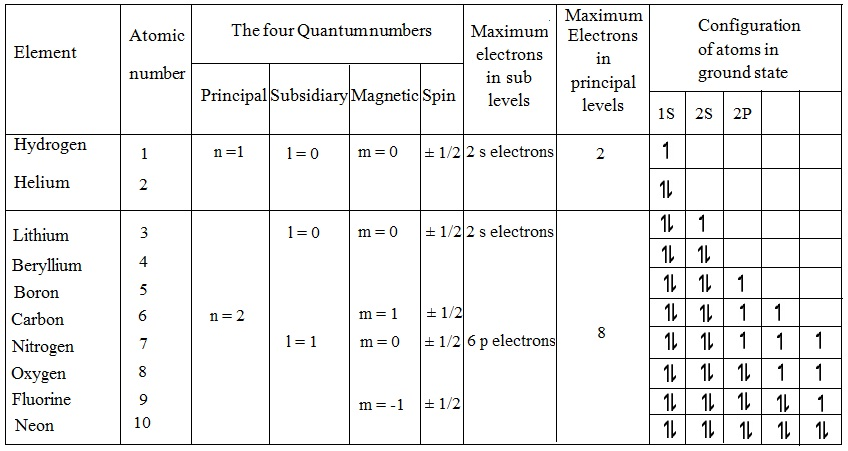#### Electronic Configuration-Atomic Orbital Model, Chemistry tutorial

Introduction:

For a long time, it was assumed that electrons were orbiting the nucleus in the similar manner the planets orbit the sun. We have learned regarding the contributions of Rutherford and Bohr to atomic structure in order to get a model of the atom. Their contributions went a long way to describe some of the observation regarding the atom. The Rutherford's model of an atom as comprising of a central positively charged nucleus and the negatively charged electrons a few distance away from the nucleus, is still satisfactory. Though, classical electromagnetic theory refuses the possibility of any stable electron orbits around the nucleus.

In Bohr's model of the atom, the electron was limited to being found in definite areas that is, it had to be found in that orbit and nowhere else. In the Wave Mechanics Model, though, there is a slight chance which the electron might be positioned at distances other than in the restricted orbits. In spite of this, we still accept Bohr's scheme for quantization of energy in the atom and that the lowest energy level of the atom is mainly stable state.

However Bohr's contribution was outstanding, specifically his quantization of energy, theory to describe the spectral lines for hydrogen atom; it consists of the given limitations:

1) The Bohr model failed to account for the frequencies of the spectral lines for the complex atoms other than hydrogen.

2) The model as well failed to satisfactorily describe why the Rutherford's atom didn't collapse as predicted through the theory of electromagnetic radiation.

The present day image of the atom is mainly based on wave mechanical or quantum mechanical treatment. The treatment imitates on the wave-nature of the electron and the quantization of energy in the atom. However such treatments are basically mathematical in nature, it explains the electron as point charge and that the density of the cloud at a particular point gives only the probability of determining electrons at that point.

Quantum Theory of Atomic Orbitals:

In case of quantum model, the atom is still assumed to comprise of a central nucleus and orbiting electrons however the physical picture of the atom is by far dissimilar from the models stated by Rutherford and Bohr. The quantum theory tries to comprehend how electrons are arranged in the atom based on wave and quantum mechanics treatment. The electron is visualized as a point charge. The density of this point charge differs in various locations around the nucleus and gives a measure of the probability of determining the electron at a particular point.

The region or space, around the nucleus, in which the electron in a particular energy level is most possibly or probable to be found is stated as an orbital. Therefore instead of explaining a fixed Bohr orbit in which the electrons are located, the modem theory gives a probability explanation of atomic orbitals. The outcome of the quantum mechanical treatment of the atom is illustrated below.

The principal quantum number (n):

You will remember that in the Bohr's model, each and every orbit is symbolized by the principal quantum number. This designation is retained in the quantum model however to symbolize distinct energy levels and not shells or orbits.

In another words, the quantum model recognizes various quantized energy levels around the nucleus. Each and every principal quantum number (n) corresponds to a specific energy level and consists of integral values of 1, 2, 3, 4 and so on. The principal quantum number n = 1 symbolize the lowest energy level that an electron can occupy. Electron having the largest (n) value consists of the most energy and occupies the highest energy level; and thus the most easily removable or ionisable electron. The maximum possible number of electrons in an energy level is represented by 2n2. (n ≥ 1)

The subsidiary or azimuthal quantum number, (l):

This subsidiary quantum number illustrates how many energy sub-levels are present in each and every electronic energy level. The subsidiary quantum number, l, consists of integral values ranging from 0, 1, 2... (n-1). The sub-levels having l = 0, 1, 2, 3, are generally termed to s-, p-, d- and f- sub-levels correspondingly.

The magnetic quantum number, (M):

The magnetic quantum number (m) represents the number of orbitals present in each and every sub-energy level and consists of integral values ranging from - l ...0 ....+ l.  For illustration, if l = 2, m consists of the values - 2, - 1, 0, 1, 2, that is, the l = 2 sub-level (d-sublevel) consists of five orbitals; if l = 1, m consists of the values - 1, 0, 1 that is, l = 1 sub-level (p- sub-level) consists of three orbitals (Observe the table).

The spin quantum number (s):

The spin quantum number, (s) illustrates the fact that each and every orbital can just hold a maximum of two electrons having opposite spin. Their spin states are represented by the spin quantum numbers -1/2 and +1/2.

Table: Atomic energy levels and sub-levels

 Value of n Values of l Number of sub-levels Names of the sub-levels 1 0 One s 2 0 and 1 Two s and p 3 0, 1 and 2 Three s, p and d 4 0, 1, 2 and 3 Four s, p, d and f

Shape of Atomic Orbitals:

On contrast to Bohr's postulate, electrons don't move around the nucleus in definite paths; however it is not possible to precisely find out the path of the electron. Instead, the location of electron is stated in terms of probabilities which are illustrated by the orbital. A region in space where there is a high probability of determining an electron in an atom is termed as an orbital. The density cloud of electrons states the shape of the orbital.

The electrons which move about to form a spherical symmetrical cloud around the nucleus is an s-electron residing in the s-orbital. The p-electrons move about three axes, x, y and z that is at right angles to one other, generating a dumb-bell cloud around the nucleus all along each axes. They are termed as the p-orbitals and are differentiated from one other by Px, Py and Pz in line by the direction of the electron cloud. The figure shown below illustrates the shapes of S, Px, Py and Pz orbitals. The geometrical representation of the d and f orbitals are more complicated and beyond the scope of this program.Fig: Geometrical representation of s- and p- orbitals

Electronic Configuration of Atoms - Orbital Model:

The quantum treatment mainly deals by the arrangement of electrons in atoms. Though, before we can apply the quantum numbers to deduce the electronic configuration of atoms, there are two significant rules that you must be well-known with.

1) Pauli Exclusion Principle defines that two electrons in the similar atom can't have the similar values for all the four quantum numbers. The principle simply signifies two electrons in an atom can't behave in a similar way.

2) Hund's Rule; defines that, in the filling of orbitals, electrons engage each energy level singly prior to electron pairing occurs.

The manner in which electrons are arranged in an atom is found out by the order of the sub-levels on a scale of increasing energy level. This is so as electrons are found in the lowest possible energy level, the ground state that is the most stable state of an atom. A simple representation of the orbitals on an energy scale is symbolized in the figure given below:1s < 2s < 2p < 3s < 3p < 4s < 3d < 4p < 4f - increasing energy

Fig: Atomic orbitals on an energy scale

The maximum number of electrons in each and every sub-level is found out by the number of orbitals present in the sub-level bearing in mind that each and every orbital can take a maximum of two electrons having opposite spin. Table represents the number of orbitals and maximum number of electrons in each and every sub-level.

Table: The number of orbitals and maximum electrons in the sub-level

 Value of l Name of sub-level Values of m Number of values of m Number of orbitals Maximum number of electrons 0 s 0 One One 2 1 p 1, 0, -1 Three Three 6 2 d 2, 1, 0, -1, -2 Five Five 10 3 f 3, 2, 1, 0, -1, -2, -3 Seven Seven 14

Beginning with hydrogen, which consists of an atomic number of 1, the electron occupies the 1s sub-level and this procedure of electronic occupation continues by the increased atomic number according to the order of increasing orbital energy levels. To carry on a check on the spin of the electron, arrows of opposite spins are employed to differentiate two electrons in an orbital. The table represents the electronic configuration of the first 10 elements by using the four quantum numbers.

Table: Electronic configurations of the atoms of elements using the four quantum numbers.Electronic Configuration and Periodic Classification of Elements:

One of the benefits of the electronic configuration of elements by employing quantum numbers is that it illustrated the basis for the periodic categorization of element. In another words, the key to the periodicity of elements lies in the electronic configurations of their atoms.

The orbital arrangement of electrons clearly exhibited that the great worth of the Period Table as it describes the groups and characteristic properties of elements.

Tutorsglobe: A way to secure high grade in your curriculum (Online Tutoring)